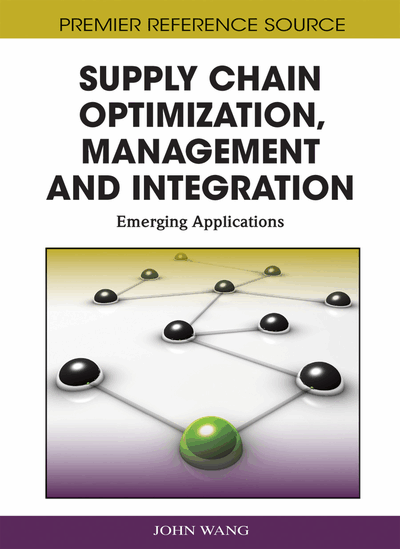# A Stochastic Perturbation Algorithm for Inventory Optimization in Supply Chains

Liya Wang (NASA Ames Research Park, USA) and Vittal Prabhu (Penn State University, USA)
DOI: 10.4018/978-1-60960-135-5.ch012

## Abstract

In recent years, simulation optimization has attracted a great deal of attention because simulation can model the real systems in fidelity and capture complex dynamics. Among numerous simulation optimization algorithms, Simultaneous Perturbation Stochastic Approximation (SPSA) algorithm is an attractive approach because of its simplicity and efficiency. Although SPSA has been applied in several problems, it does not converge for some. This research proposes Augmented Simultaneous Perturbation Stochastic Approximation (ASPSA) algorithm in which SPSA is augmented to include presearch, ordinal optimization, non-uniform gain, and line search. Performances of ASPSA are tested on complex discrete supply chain inventory optimization problems. The tests results show that ASPSA not only achieves speed up, but also improves solution quality and converges faster than SPSA. Experiments also show that ASPSA is comparable to Genetic Algorithms in solution quality (6% to 15% worse) but is much more efficient computationally (over 12x faster).
Chapter Preview
Top

## Augmented Simulataneous Perturbation Stochastic Approximation (Aspsa) Algorithm

The list of notations we will use in the paper is as following:

• i: Index of decision variable

• θ: Decision vector

• p: Dimension of decision vector

• k: Iteration index

• yk: Noisy objective

• Δk: Perturbation vector

• n: Full simulation run length

• ns: Shorter simulation run length (ns < n)

• m: Pre-search number

• l: Line search number

## Complete Chapter List

Search this Book:
Reset